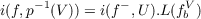#### Vol. 21, No. 3, 1967

 Download this articleFor screen For printingRecent Issues Vol. 325: 1  2 Vol. 324: 1  2 Vol. 323: 1  2 Vol. 322: 1  2 Vol. 321: 1  2 Vol. 320: 1  2 Vol. 319: 1  2 Vol. 318: 1  2Online Archive Volume: Issue:The Journal Subscriptions Editorial Board Officers Contacts Submission Guidelines Submission Form Policies for Authors ISSN: 1945-5844 (e-only) ISSN: 0030-8730 (print) Special Issues Author Index To Appear Other MSP Journals
Fixed points and fibre

### Robert F. Brown

Vol. 21 (1967), No. 3, 465–472
##### Abstract

Let = (E,p,B) be a (Hurewicz) fibre space and let λ be a lifting function for For W a subset of B, a map f : p1(W) E is called a fibre map if p(e) = p(e) implies p(f(e)) = p(f(e)). Define f : W B to be the map such that fp = pf. If [W f(W)]V B where V is pathwise connected, define fbV : p1(b) p1(b), for b W, by fbV (e) = λ(f(e))(1) where ω;I V is a path such that ω(0) = f(b)and ω(1) = b. Let i be a fixed point index defined on the category of compact ANR’s and let Q denote the rationals. The main result of this paper is: THEOREM 1. Let = (E,p,B) be a fibre space such that E,B, and all the fibres are compact ANR’s. Let f : E E be a fibre map. If U is an open subset of B such that f(b)b for all b bd(U) and cl [U f(U)] V where V is open and pathwise connected and ℱ|V = (p1(V ),p,V ) is Q-orientable, thenwhere L(fbV ) is the Lefschelz number of fbV for any b U.

Primary: 55.50
##### Milestones